# NCERT Solutions class-11 Maths Exercise 3.1## myCBSEguide App

CBSE, NCERT, JEE Main, NEET-UG, NDA, Exam Papers, Question Bank, NCERT Solutions, Exemplars, Revision Notes, Free Videos, MCQ Tests & more.

Exercise 3.1

1. Find the radian measures corresponding to the following degree measures:

(i)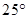(ii)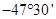(iii)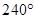(iv)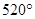Ans. (i)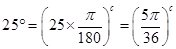(ii)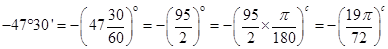(iii)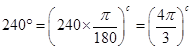(iv)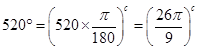2. Find the degree measures corresponding to the following radian measures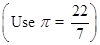.

(i)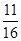(ii)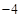(iii)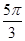(iv)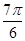Ans. (i)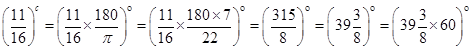=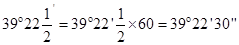(ii)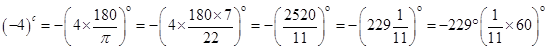=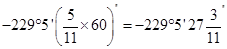(iii)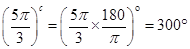(iv)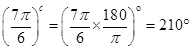3. A wheel makes 360 revolutions in one minute. Through how many radians does it turn in one second?

Ans. Number of revolutions in 1 minute = 360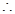Number of revolution in 60 seconds = 360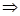Number of revolutions in 1 second =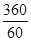= 6 revolutionsAngle made by wheel in 6 revolutions =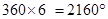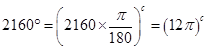4. Find the degree measure of the angle subtended at the centre of a circle of radius 100 cm by an arc of length 22 cm.

Ans. Here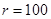cm and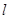= 22 cm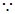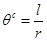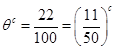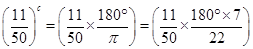=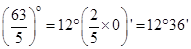5. In a circle of diameter 40 cm, the length of a chord is 20 cm. Find the length of minor arc of the chord.

Ans. Given: Diameter AB = 40 cm, Radius OA = 20 cm and Chord AC = 20 cm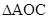is an equilateral triangle.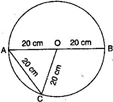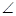AOC =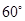=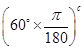=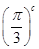Now,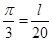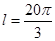cm

6. If in two circles, arcs of the same length subtend anglesand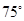at the centre, find the ratio of their radii.

Ans. Let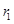and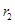be radii of two circles in which arcs of same lengthsubtend angles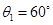and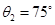respectively.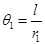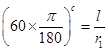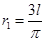And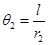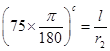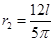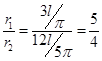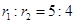7. Find the angle in radians through which a pendulum swings if its length is 75 cm and the tip describes an arc of length:

(i) 10 cm

(ii) 15 cm

(iii) 21 cm

Ans. (i) Given: length of pendulum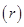= 75 cm and length of arc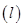= 10 cm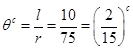(ii) Given: length of pendulum= 75 cm and length of arc= 15 cm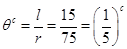(iii) Given: length of pendulum= 75 cm and length of arc= 21 cm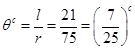### 1 thought on “NCERT Solutions class-11 Maths Exercise 3.1”

1. I really understand this chapter